Disclosure: This post may contain affiliate links, meaning when you click the links and make a purchase, we receive a commission.

# How to Show Formula in Excel Cells Instead of Value (6 Ways)

In Excel, a lot of things are done using the functions that together make formulas. In this article, we are going to show you some tips on “How to Show Formula in Excel Cells Instead of Value”. Sometimes while performing a task in Excel you are more concerned with the formulas than the results. Hopefully, after reading this article you will get a number of ways of doing that.

Suppose you have calculated areas for some given data where the heights and widths are given. The formula for calculating the area is, Area= Height*Width. Here the heights and widths are given in certain cells. Suppose you calculated the area using the formula =A2*B2. Where the height is in cell A2 and width is in cell B2. Instead of showing the results we will only show the associated formula for these.

## Clicking Show Formulas from Formulas Tab

This is the best way for showing the formulas in Excel. Let`s say your worksheet looks like the picture below where you calculated the areas based on some heights and widths.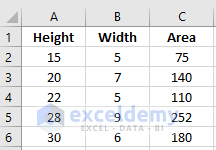The procedure for this is given below.

• Click on to the Formulas tab on top of your Excel worksheet.
• Under the Formula Auditing Section click on the Show Formulas option.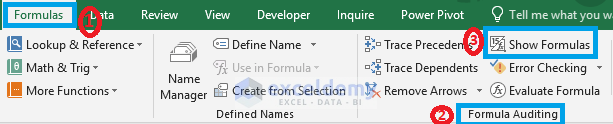• After you click the Show Formulas option you will get to see the below result where the formulas will be shown instead of the values in the formulated cells.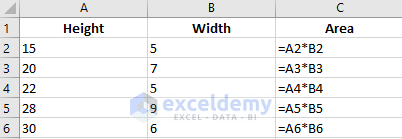This is kind of a long process and after doing this you will always get to see the formulas instead of values. The process is stated below.

• Click on to the File tab on top of your Excel worksheet.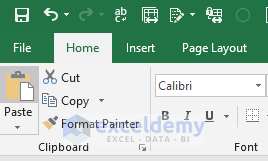• After this click on to the Options section below.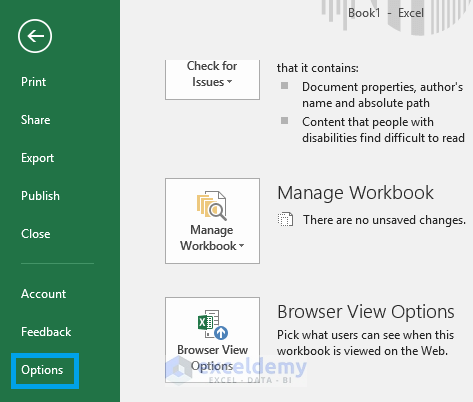• In the Excel Options dialogue box, click on to the Advanced option and scroll downwards until you get the Display options for this worksheet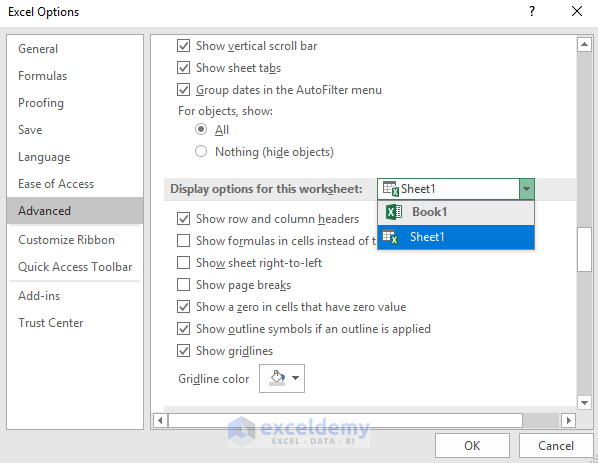• Now in the Display options for this worksheet select the worksheet where you want the formulas to be displayed and click on to the Show formulas in cells instead of their calculated results. After clicking this press OK.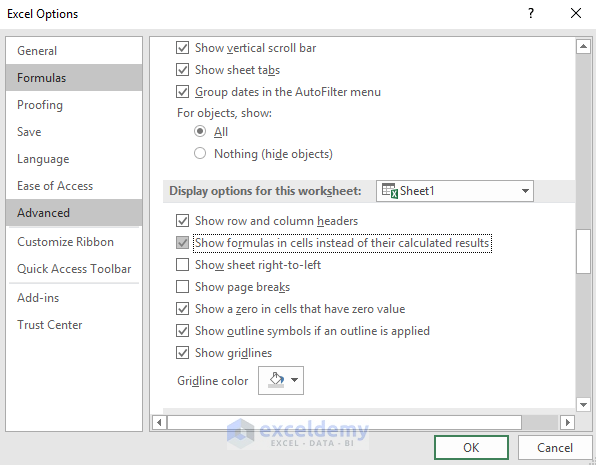• This will give you the below result. Now, whenever you are going to insert a formula for getting a result it will only show you the formula instead of values.## Using Keyboard Shortcut

The fastest way of showing formula instead of values is by using the keyboard shortcut. In anywhere of your Excel worksheet press Ctrl + ` together. You will see that the values that are found by using formulas are turned into formulas. This is because on those cells those formulas were applied. Press Ctrl+` again if you want to disable this feature.

## Using ‘’=’ Instead of “=” while applying Formula

Suppose you have your worksheet with formula applied in it and you want some or let`s say one of your cell to show you the formula that has been applied. To do that, click on that specific cell and insert the grave sign “`” just before the equal sign.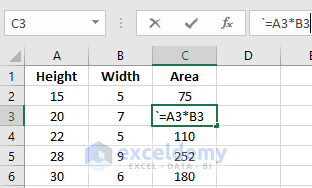## Replacing “=” with “’=”

We all know that Excel Formula starts with “=” sign. Now if you insert anything before the equal sign the formula will not be applied. Usually, the grave signs are used ahead of the equal sign to show formulas. If you applied formulas in your worksheet and somehow you want some or every formulated cell of your worksheet to show the formulas you can replace the “=” sign with a “`=” sign. To perform this press Ctrl + H together. You will get a Find and Replace dialogue box. In that dialogue box do the followings and press onto the Replace All option. You will get to see all of your formulas by doing this.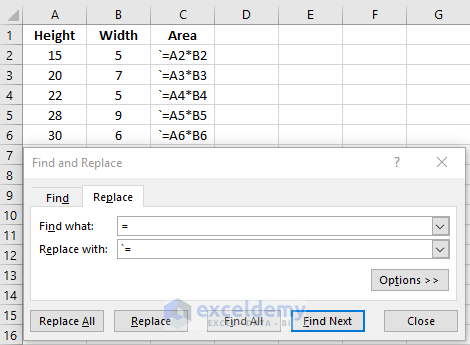## Using the Format Cells Option

If you make the formatting of the formulated cells as the Text format you can easily show the formulas instead of values. For doing this select the formulated cells of your worksheet and right click on your mouse button to select the Format Cells option.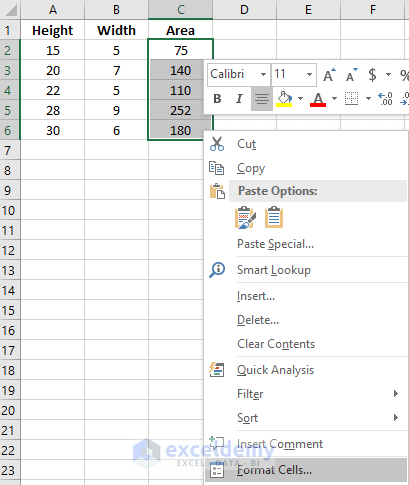Under the Format Cells option select the Text option and press OK.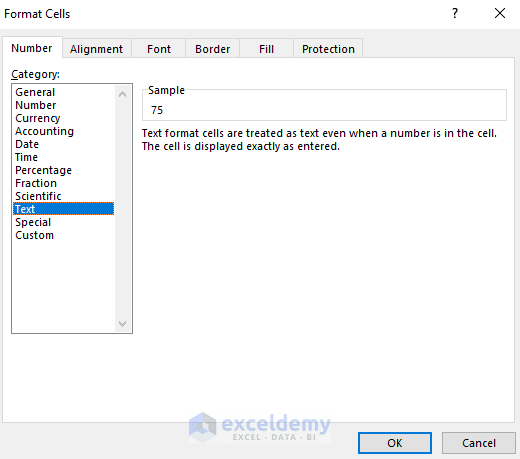Initially, you will not see the formulas. Click on those formulated cells and on the Formula bar press Enter. You will see that instead of values you are now getting the formulas.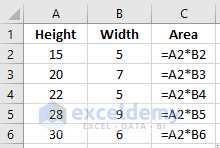Note: This is not a good practice of showing formulas as after the formatting if you need to convert your formulas as the values again you again need to format them as General or Numbers.

## Conclusion

As you can see we have demonstrated so many methods on how to show formula in Excel cells instead of value. You can choose any option you like. In my opinion, using the Keyboard Shortcut and Clicking Show Formulas from Formulas Tab are the best options among these. Hope you will like this article. Don’t hesitate to ask us any questions. Cheers.

## Related Articles

1. How to Use the FORMULATEXT Function in Excel
Hello!

Welcome to my Profile. Here I will be posting articles related to Microsoft Excel. I have completed my BSc in Electrical and Electronic Engineering from American International University-Bangladesh. I am a diligent, goal-oriented engineer with an immense thirst for knowledge and attitude to grow continuously. Continuous improvement and life-long learning is my motto.

We will be happy to hear your thoughts